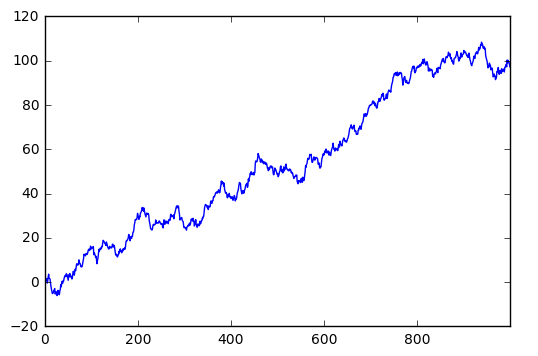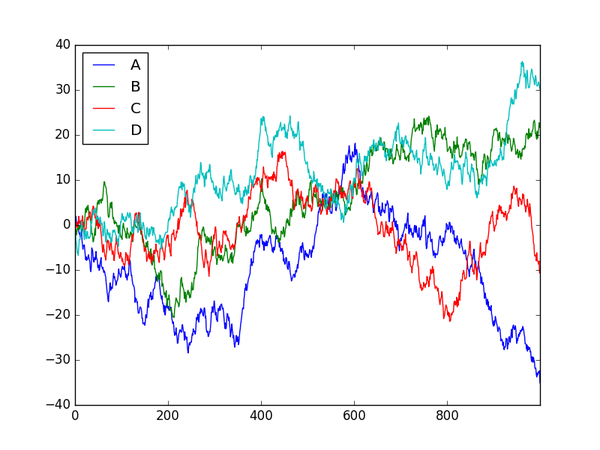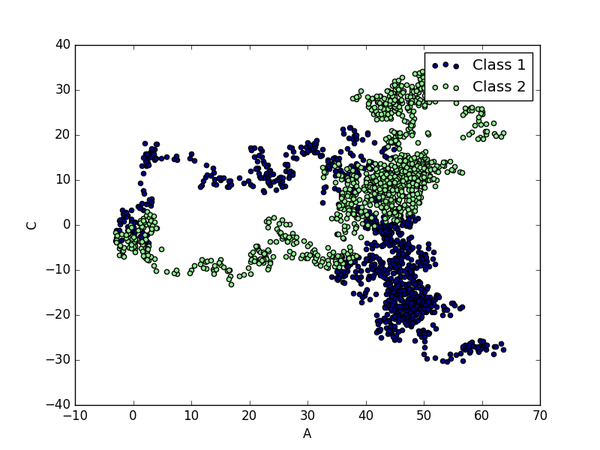# Pandas plot 出图

673℃

``pip install matplotlib``

``````import pandas as pd
import numpy as np
import matplotlib.pyplot as plt``````

## 创建一个Series

``````# 随机生成1000个数据
data = pd.Series(np.random.randn(1000),index=np.arange(1000))

# 为了方便观看效果, 我们累加这个数据
data.cumsum()

# pandas 数据可以直接观看其可视化形式
data.plot()

plt.show()``````## Dataframe 可视化

``````data = pd.DataFrame(
np.random.randn(1000,4),
index=np.arange(1000),
columns=list("ABCD")
)
data.cumsum()
data.plot()
plt.show()``````• bar
• hist
• box
• kde
• area
• scatter
• hexbin

``ax = data.plot.scatter(x='A',y='B',color='DarkBlue',label='Class1')``

``````# 将之下这个 data 画在上一个 ax 上面
data.plot.scatter(x='A',y='C',color='LightGreen',label='Class2',ax=ax)
plt.show()``````